# Flip-flop types, their Conversion and Applications

Flip-flop is a circuit that maintains a state until directed by input to change the state. A basic flip-flop can be constructed using four-NAND or four-NOR gates. Flip flop is popularly known as the basic digital memory circuit. It has its two states as logic 1(High) and logic 0(low) states. A flip flop is a sequential circuit which consist of single binary state of information or data. The digital circuit is a flip flop which has two outputs and are of opposite states. It is also known as a Bistable Multivibrator.

Types of flip-flops:

1. SR Flip Flop
2. JK Flip Flop
3. D Flip Flop
4. T Flip Flop

Logic diagrams and truth tables of the different types of flip-flops are as follows:

### S-R Flip Flop :

In the flip flop, with the help of preset and clear when the power is switched ON, the states of the circuit keeps on changing, that is it is uncertain. It may come to set(Q=1) or reset(Q’=0) state. In many applications, it is desired to initially set or reset the flip flop that is the initial state of the flip flop that needs to be assigned. This thing is accomplished by the preset(PR) and the clear(CLR).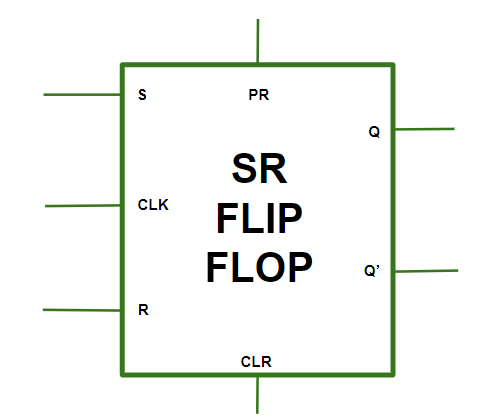S-R Flip Flop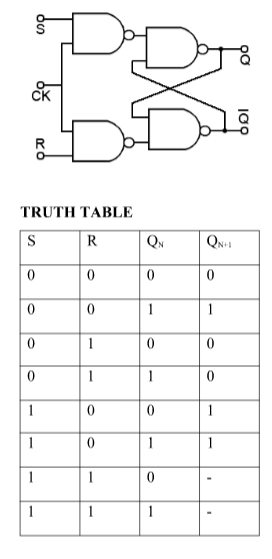### Operations:

Case 1:

PR=CLR=1 The asynchronous inputs are inactive and the flip flop responds freely to the S,R and the CLK inputs in the normal way.

Case 2:

PR=0 and CLR=1 This is used when the Q is set to 1.

Case 3:

PR=1 and CLR=0 This is used when the Q’ is set to 1.

Case 4:

PR=CLR=0 This is an invalid state.

Characteristics Equation for SR Flip Flop: QN+1 =  QNR’ + SR’

### J-K Flip Flop:

In JK flip flops, the diagram over here represents the basic structure of the flip flop which consists of Clock (CLK), Clear (CLR), Preset (PR).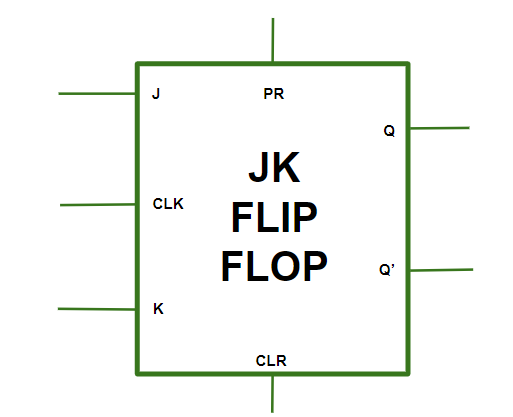J-K Flip Flop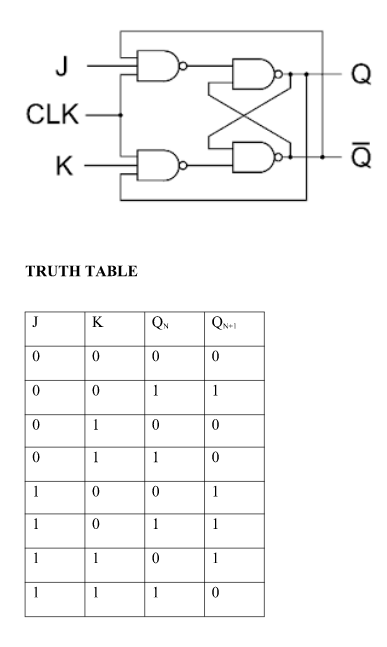### Operations:

Case 1:

PR=CLR=0 This condition is in its invalid state.

Case 2:

PR=0 and CLR=1 The PR is activated which means the output in the Q is set to 1. Therefore, the flip flop is in the set state.

Case 3:

PR=1 and CLR=0 The CLR is activated which means the output in the Q’ is set to 1. Therefore, the flip flop is in the reset state.

Case 4:

PR=CLR=1 In this condition the flip flop works in its normal way whereas the PR and CLR gets deactivated.

### Race around condition:

When the J and K both are set to 1, the input remains high for a longer duration of time, then the output keeps on toggling. Toggle means that switching in the output instantly i.e. Q=0, Q’=1 will immediately change to Q=1 and Q’=0 and this continuation keeps on changing. This change in output leads to race around condition.

Characteristics Equation for JK Flip Flop: QN+1 = JQ’N + K’QN

### D Flip Flop: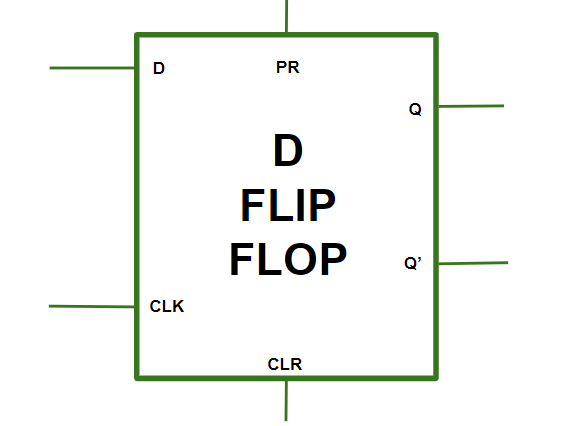D FLIP FLOP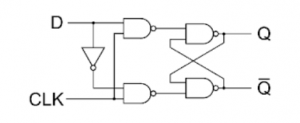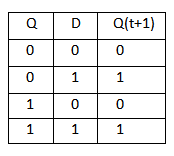Characteristics Equation for D Flip Flop: QN+1 = D

### T Flip Flop: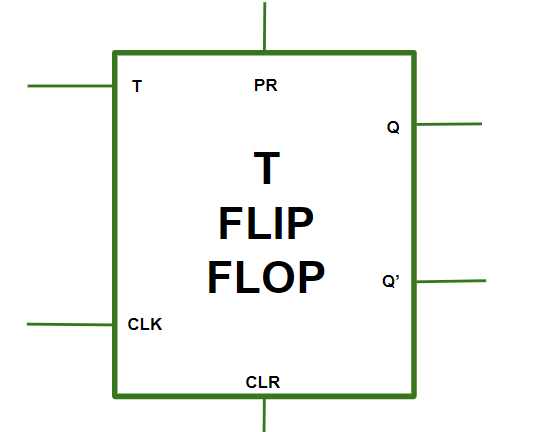T FLIP FLOP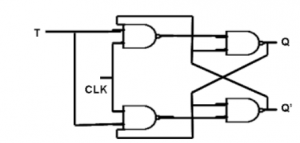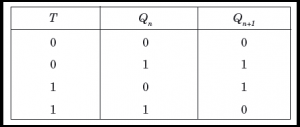Characteristics Equation for T Flip Flop: QN+1 = Q’NT + QNT’ = QN XOR T

### Conversion for Flip Flops:

EXCITATION TABLE: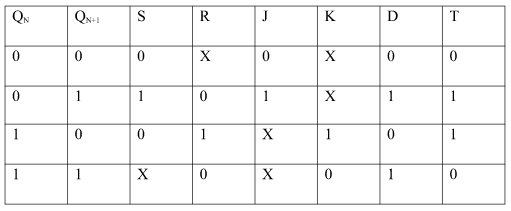Steps To Convert from One Flip Flop to Other :

Let there be required flipflop to be constructed using sub-flipflop:

1. Draw the truth table of the required flip-flop.
2. Write the corresponding outputs of sub-flipflop to be used from the excitation table.
3. Draw K-Maps using required flipflop inputs and obtain excitation functions for sub-flipflop inputs.
4. Construct a logic diagram according to the functions obtained.

i) Convert SR To JK Flip Flop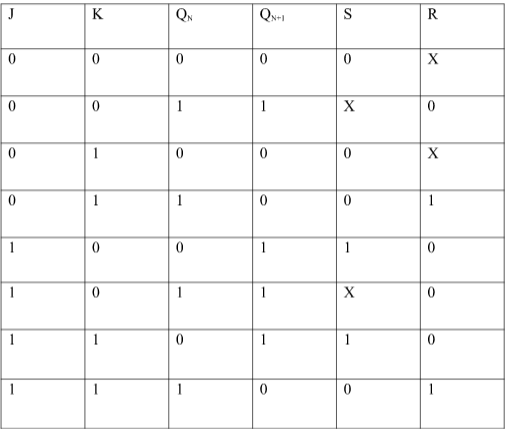Excitation Functions: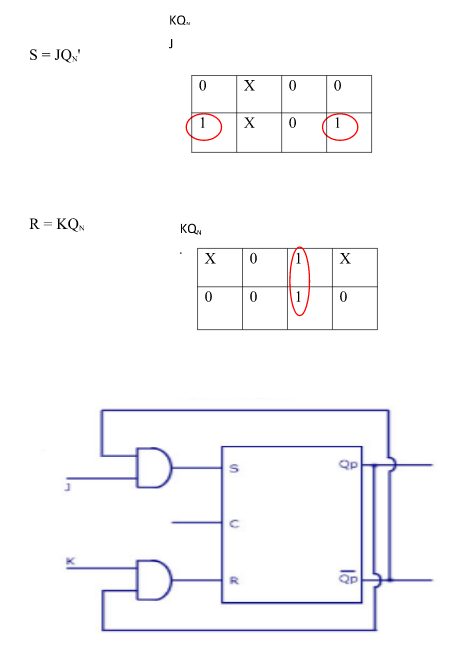ii) Convert SR To D FlipFlop: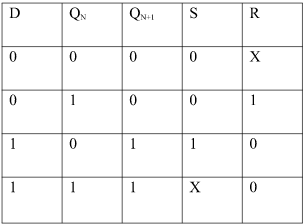Excitation Functions: S = D, R = D

###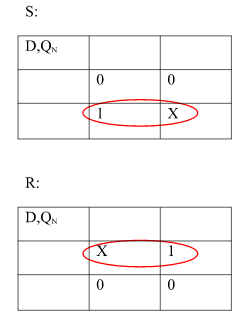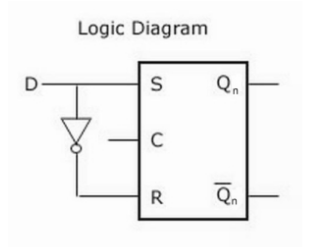Applications of Flip-Flops:

These are the various types of flip-flops being used in digital electronic circuits and the applications of Flip-flops are as specified below.

• Counters
• Frequency Dividers
• Shift Registers
• Storage Registers
• Bounce elimination switch
• Data storage
• Data transfer
• Latch
• Registers
• Memory